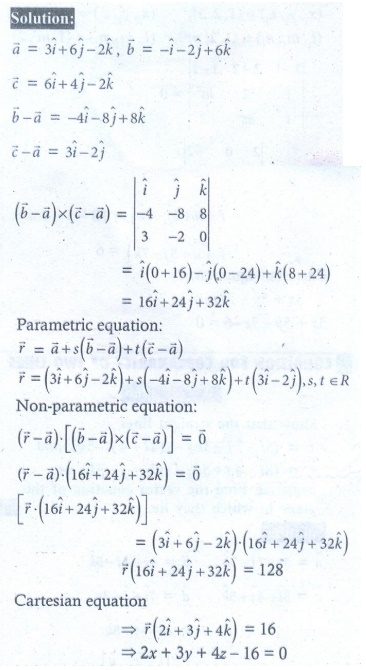Home | | Maths 12th Std | Exercise 6.7: Equation of a plane

# Exercise 6.7: Equation of a plane

Maths Book back answers and solution for Exercise questions - Mathematics : Equation of a plane Questions with Answer

EXERCISE 6.7

1. Find the non-parametric form of vector equation, and Cartesian equation of the plane passing through the point (2, 3, 6) and parallel to the straight lines2. Find the parametric form of vector equation, and Cartesian equations of the plane passing through the points (2, 2,1), (9,3, 6) and perpendicular to the plane 2+ 6 6= 9 .3. Find parametric form of vector equation and Cartesian equations of the plane passing through the points (2, 2,1), (1, -2, 3) and parallel to the straight line passing through the points (2,1, -3) and (-1,5, -8) .4. Find the non-parametric form of vector equation and cartesian equation of the plane passing through the point (1, -2, 4) and perpendicular to the plane x + 2 y - 3z = 11 and parallel to the line.5. Find the parametric form of vector equation, and Cartesian equations of the plane containing the line= (ˆ- ˆ+ 3ˆ) + t(2ˆ- ˆ+ 4ˆ) and perpendicular to plane= (iˆ + 2ˆ + ˆ) = 86. Find the parametric vector, non-parametric vector and Cartesian form of the equations of the plane passing through the three non-collinear points (3, 6, -2),(-1, -2, 6) , and (6, -4, -2) .7. Find the non-parametric form of vector equation, and Cartesian equations of the plane